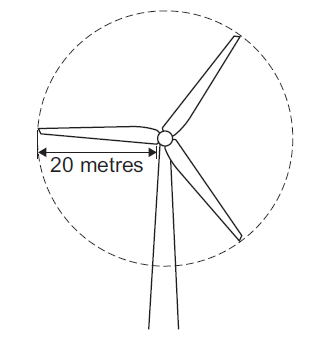# Energy and Work Done – Question 2

The diagram shows a wind turbine.(a) The blades of the turbine are 20 metres long. On average, 15 000 kg of air, moving at a speed of 12 m/s, hit the blades every second.

Using an appropriate equation, calculate the kinetic energy of the air hitting the blades
every second.Kinetic energy = ......................... J
(2 marks)

-

(b) Part of the kinetic energy of the wind is transformed into electrical energy.

The diagram shows that, for the same wind speed, the power output of a turbine, in kilowatts, depends on the length of the turbine blades.Give a reason why doubling the diameter of the blades more than doubles the power output of a turbine.(1 mark)

-

How did you do? Click to view the Mark Scheme AnswersFrom Q4 P2 AQA January 2011 (Higher)

Energy and Work Done – Question 2 was last modified: November 18th, 2015 by